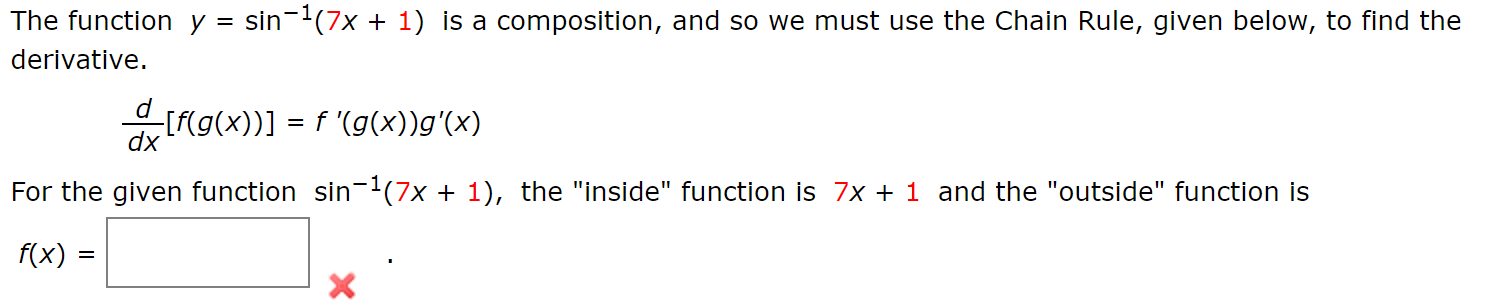# sin (7x The function y 1) is a composition, and so we must use the Chain Rule, given below, to find the = derivative [f(g(x))] f (g(x))g'(x) dx For the given function sin-2(7x + 1), the "inside" function is 7x 1 and the "outside" function is f(x) = X

Questionhelp_outlineImage Transcriptionclosesin (7x The function y 1) is a composition, and so we must use the Chain Rule, given below, to find the = derivative [f(g(x))] f (g(x))g'(x) dx For the given function sin-2(7x + 1), the "inside" function is 7x 1 and the "outside" function is f(x) = X fullscreen